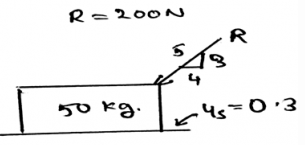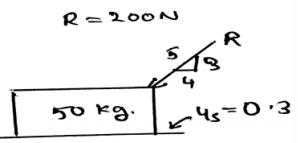Courses

# Test: Problems Involving Dry Friction

## 15 Questions MCQ Test Engineering Mechanics - Notes, Videos, MCQs & PPTs | Test: Problems Involving Dry Friction

Description
This mock test of Test: Problems Involving Dry Friction for Mechanical Engineering helps you for every Mechanical Engineering entrance exam. This contains 15 Multiple Choice Questions for Mechanical Engineering Test: Problems Involving Dry Friction (mcq) to study with solutions a complete question bank. The solved questions answers in this Test: Problems Involving Dry Friction quiz give you a good mix of easy questions and tough questions. Mechanical Engineering students definitely take this Test: Problems Involving Dry Friction exercise for a better result in the exam. You can find other Test: Problems Involving Dry Friction extra questions, long questions & short questions for Mechanical Engineering on EduRev as well by searching above.
QUESTION: 1

### What does the Newton’s third law states?

Solution:

The requirement of the third law is important in the making of the equilibrium equations of the body. The body particles are in the equilibrium and are thus facing the forces and to be in the equilibrium they also react and apply the opposite force and thus the third law of Newton. The forces here can be normal forces or the loading forces.

QUESTION: 2

### The net force of the body is zero that means the force are not being applied to the body at all and hence the body is in equilibrium.

Solution:

The net force of the body is zero that doesn’t means that the force are not being applied to the body at all and hence the body is in equilibrium. The equilibrium is only attained if the net force on the body tends to be equal to zero. Thus the forces cancels out. If this happens there is no motion of the body along any direction and hence the body is said to be in equilibrium.

QUESTION: 3

### In the simplification of the forces for the free body diagram the net force acts at the ___________ of the loading body.

Solution:

QUESTION: 4

For a vector F, Fcosβ is equal to zero. What does this refer?

Solution:

As we know the α, β and γ are the angles made by the x, y and z-axis respectively. Thus y-axis component is zero, or β = 90˚. And thus if the angle is giving component to be zero this means the vector in that particular axis is perpendicular to that axis. This concept is used when the wedge is involved in the motion.

QUESTION: 5

As in the determination of the various unknown forces in the free body diagram involves the use of vectors. So one of the vector law is commutative law and it is valid for the cross product of two vectors. (Commutative law: PxQ = QxP; for two vectors P and Q)

Solution:

This statement is wrong. It is not possible, unless we apply a negative sign to the RHS of the equation. That is PxQ = -(QxP). It is because, if you curl your wrist from one vector towards another vector, the thumb projected will give the direction of the cross product. Thus if you reverse the direction, negative sign is necessary.

QUESTION: 6

As the free body diagram of the problem statement involves the use of forces. So in them the ___________ forces do not cause the rotation.

Solution:

The concurrent forces are the one are somewhere touching the axis of rotation. If any of the force is touching that axis, that force is not considered, or is insufficient to cause a rotation. If a force is concurrent then the perpendicular distance of the force from the line of axis is zero, thus no rotation. As we know rotation is caused by moment.

QUESTION: 7

For equilibrium the normal forces acts in which direction in the free body diagrams if they are constructed for the friction part calculations?

Solution:

As the loads are being acting in the downward direction. Thus to make the forces balance, the normal forces act in the vertically upward direction. As we know that when there is no lubricating fluid present between the surfaces in contact, the dry friction occurs. This friction magnitude is taken out from these normal forces.

QUESTION: 8

Which of the following is the assumption on the property of the surface on which block is present in case we are dealing with the calculations involving the friction?

Solution:

The block used to explain the theory of friction is a used in a deformable surface. As we know that the theory of friction says that when there is no lubricating fluid present between the surfaces in contact, the dry friction occurs. Thus to show the same experiments are done over a non-rigid/deformable surface.

QUESTION: 9

Calculate the Normal force developed between the body and the surface.Solution:

The net forces acting on the body is shown by the help of the resultant forces. There are two types, first the frictional and the second is the normal. This is because the resultant forces have the sum of all the forces which are acting on the direction which is same.

QUESTION: 10

There are three types of friction problems. One of them is _____________

Solution:

There are mainly three types of the friction problems. They are no-apparent impending motion, impending motion at all points of contact and impending motion at some points of contacts. Therefore the answer is impending motion at all points of contact. There is involvement of various types of calculations which is there in all of the three types.

QUESTION: 11

The phenomena of horizontal pull and push explains something related to the friction, among the following what is it?

Solution:

As we know that when there is no lubricating fluid present between the surfaces in contact, the dry friction occurs. This is the phenomena that defines that there is a resistance which is present there between the two surfaces. Thus the pull and push phenomena explains the theory of friction.

QUESTION: 12

There are main two types of forces which are being stated in the free body diagram, and they are generally the resultant of various forces which are being acted over the body. Which are they?

Solution:

The net forces acting on the body is shown by the help of the resultant forces. There are two types, first the frictional and the second is the normal. This is because the resultant forces have the sum of all the forces which are acting on the direction which is same.

QUESTION: 13

The frictional force is directly proportional to the surface of the solid.

Solution:

The frictional force is directly proportional to the vertical forces that is being applied normal to the surface of the body. The force of friction is not dependent on the type of the surface. Thus the only thing the frictional force does depend is the normal force.

QUESTION: 14

Calculate the frictional force developed between the body and the surface.Solution:

The net forces acting on the body is shown by the help of the resultant forces. There are two types, first the frictional and the second is the normal. This is because the resultant forces have the sum of all the forces which are acting on the direction which is same.

QUESTION: 15

The frictional force developed always acts ____________ to the surface of the application of the friction.

Solution:

The friction is the phenomena that defines that there is a resistance which is present there between the two surfaces. This friction is applied tangentially to the surfaces in contact. Thus the main thing is that the forces on both of the surfaces act tangential to each other.